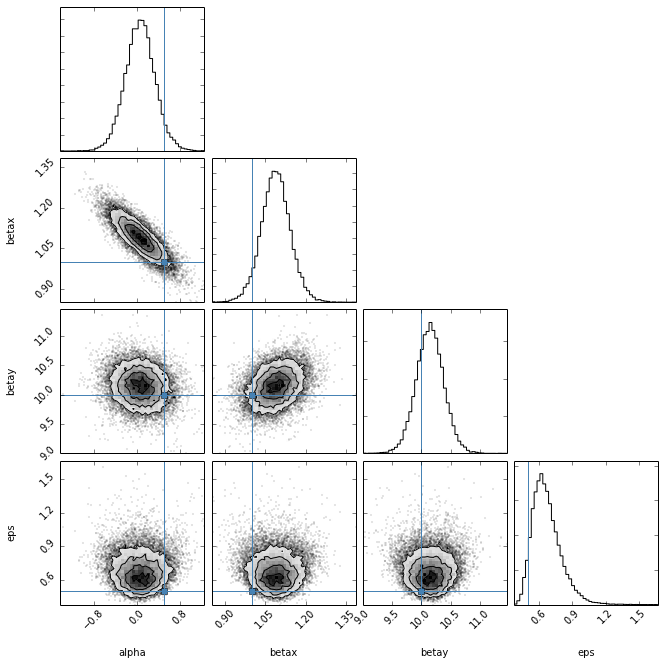PyStan Example¶

PyStan is a python interface to STAN, a C++ library for building Bayesian models and sampling them with Markov Chain Monte Carlo (MCMC). To install pystan, you'll need to install cython. Then download the pystan package from:

https://github.com/stan-dev/pystan

You could also try to install via pip or easy_install, but I had problems using those and could only compile from source.

Similar to previous examples, we start by making some fake data. In this case, we're going to do have a linear regression model with two predictor variables (x,y) and one outcome variable (z). The relation between them is: $Z_n = \alpha + \beta_x x_n + \beta_y y_n + \epsilon$ where $\epsilon$ is a Gaussian noise term.

In :
from numpy import *
Nobs = 20
x_true = random.uniform(0,10, size=Nobs)
y_true = random.uniform(-1,1, size=Nobs)
alpha_true = 0.5
beta_x_true = 1.0
beta_y_true = 10.0
eps_true = 0.5
z_true = alpha_true + beta_x_true*x_true + beta_y_true*y_true
z_obs = z_true + random.normal(0, eps_true, size=Nobs)

We can plot the data through two diferent slices of the data. We'll color code each point by it's value of the other predictor to see the trend in both cases.
In :
%matplotlib inline
from matplotlib import pyplot as plt
plt.figure(figsize=(12,6))
plt.subplot(1,2,1)
plt.scatter(x_true, z_obs, c=y_true, marker='o')
plt.colorbar()
plt.xlabel('X')
plt.ylabel('Z')
plt.subplot(1,2,2)
plt.scatter(y_true, z_obs, c=x_true, marker='o')
plt.colorbar()
plt.xlabel('Y')
plt.ylabel('Z')

Out:
<matplotlib.text.Text at 0x10c8e5a10>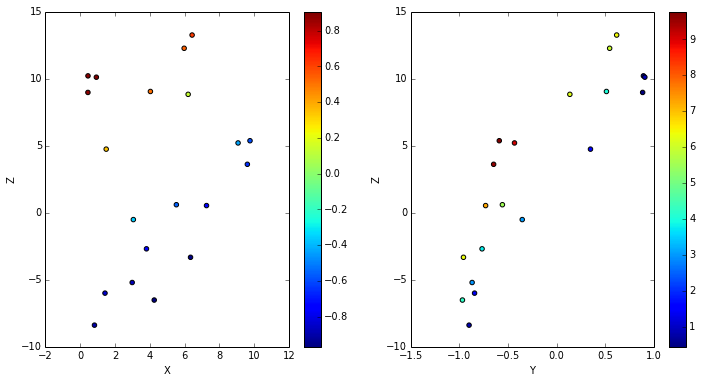Now we build the STAN model that we are going to use for this data. STAN is a different language (similar to C), so we have to put it in a string, or put it in a file.

In :
model = """
data {
int<lower=4> N; // Number of data points
real x[N];      // the 1st predictor
real y[N];      // the 2nd predictor
real z[N];      // the outcome
}
parameters {
real alpha;     // intercept
real betax;     // x-slope
real betay;     // y-slope
real<lower=0> eps;       // dispersion
}
model {
for (i in 1:N)
z[i] ~ normal(alpha + betax * x[i] + betay * y[i], eps);
}"""


The STAN model has three sections in this case. The first defines the data that will be fit. Data types can be integers (int) or floating-point numbers (real). They can also have contraints. Above, we specify that N > 0. Next, we define the parameters of the model. Then, we define the model section that defines the likelihood. In this case, it is assumed that each data point was drawn from a normal distribution with mean equal to the regression model and standard deviation equal to eps. We put it in a for loop over all the data points.

With this model, we can then fit the data using pystan. But first, we need to put the data into a form that pystan can use. We do this by making a dictionary with key equal to the variable name and value equal to the data.

In :
data = {'N':Nobs, 'x':x_true, 'y':y_true, 'z':z_obs}

Now, we give it all to pystan and fit the data. Yes, it's really that easy.
In :
import pystan
fit = pystan.stan(model_code=model, data=data, iter=1000, chains=4)

INFO:pystan:NOW ON CHAIN 0
INFO:pystan:NOW ON CHAIN 1
INFO:pystan:NOW ON CHAIN 2
INFO:pystan:NOW ON CHAIN 3



We give stan the model string and the data. We ask for 1000 MCMC iterations each run on 4 parallel chains. The first time you fit a model, pystan converts the STAN model into C++ code (making it fast), so it make take a while to start up. Once it's done, we can get a summary of the results:

In :
print fit

Inference for Stan model: anon_model_a87850813aab96ee1be5112f40cf12e0.
4 chains, each with iter=1000; warmup=500; thin=1;
post-warmup draws per chain=500, total post-warmup draws=2000.

mean se_mean   sd 2.5%  25%  50%  75% 97.5% n_eff Rhat
alpha  0.0     0.0  0.3 -0.5 -0.1  0.0  0.2   0.6 772.0  1.0
betax  1.1     0.0  0.1  1.0  1.1  1.1  1.1   1.2 672.0  1.0
betay 10.1     0.0  0.2  9.7 10.0 10.1 10.3  10.6 187.0  1.0
eps    0.7     0.0  0.1  0.5  0.6  0.7  0.8   1.0 610.0  1.0
lp__  -1.7     0.1  1.6 -5.8 -2.5 -1.3 -0.5   0.3 180.0  1.0

Samples were drawn using NUTS(diag_e) at Sat Aug  2 23:10:16 2014.
For each parameter, n_eff is a crude measure of effective sample size,
and Rhat is the potential scale reduction factor on split chains (at
convergence, Rhat=1).



This will show you some summary statistics. We can always perform our own using numpy functions. We can also plot out the chains and posterior probability distributions.

In :
p = fit.plot()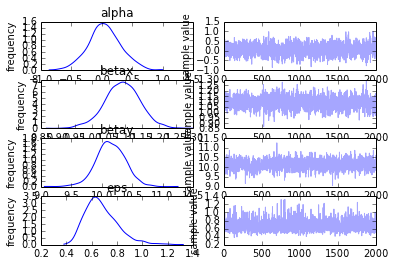You can also run the MCMC sampler for longer. This should be faster because the model is already set up.

In :
fit2 = pystan.stan(fit=fit, data=data, iter=10000, chains=4)
temp = fit2.plot()

INFO:pystan:NOW ON CHAIN 0
INFO:pystan:NOW ON CHAIN 1
INFO:pystan:NOW ON CHAIN 2
INFO:pystan:NOW ON CHAIN 3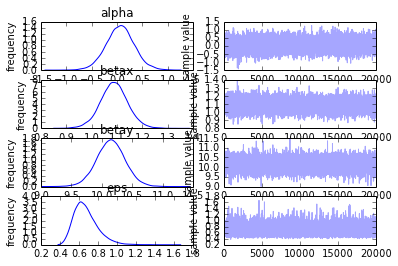And of course, you can always plot the results to see how they look. But this time, we can correct for the other predictor's effect. First, let's get the median of each variable from the samples. There are two ways to extract the chains: 1) using permuted=True to get a dictionary with each key corresponding to a parameter, or 2) using permuted=False, which returns a 3D array with indeces (i,c,p), corresponding to iteration i in chain c for parameter p.

In :
samples = fit2.extract(permuted=True)
alpha = median(samples['alpha'])
beta_x = median(samples['betax'])
beta_y = median(samples['betay'])
eps = median(samples['eps'])


The median is more robust toward non-symmetric distributions (such as the one for eps). Now we can plot the results, this time correcting for the y predictor when plotting versus x and vice versa. That way we can make sure the correct trend has been found. We can also plot a shaded region denoting the intrinsic dispersion.

In :
plt.figure(figsize=(12,6))
plt.subplot(1,2,1)
plt.plot(x_true, z_obs-alpha-beta_y*y_true, 'o')
plt.xlabel('X')
plt.ylabel('Z - alpha - beta_y y')
# Now plot the model
xx = array([x_true.min(), x_true.max()])
plt.plot(xx, xx*beta_x)
plt.plot(xx, xx*beta_x + eps, '--', color='k')
plt.plot(xx, xx*beta_x - eps, '--', color='k')
plt.subplot(1,2,2)
plt.plot(y_true, z_obs-alpha-beta_x*x_true, 'o')
plt.xlabel('Y')
plt.ylabel('Z - alpha - beta_x x')
yy = array([y_true.min(), y_true.max()])
plt.plot(yy, yy*beta_y)
plt.plot(yy, yy*beta_y + eps, '--', color='k')
plt.plot(yy, yy*beta_y - eps, '--', color='k')

Out:
[<matplotlib.lines.Line2D at 0x112c901d0>]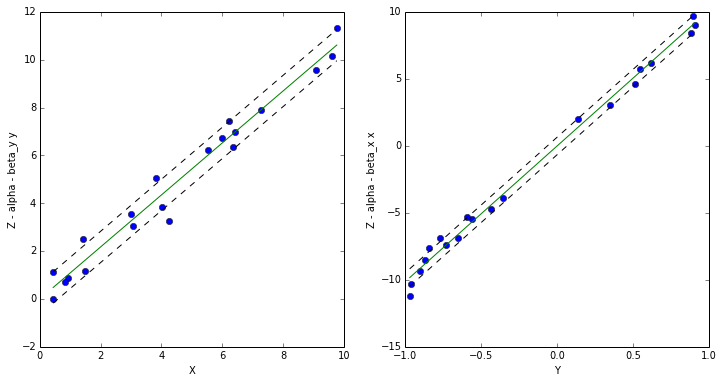If you have the triangle_plot plotting package, it's an easy way to look at the covariance of the various parameters. For this to work, we need the Markov chains in a 2D array indexed by [i,p], so we need to reshape the array to combine the 4 chains. pystan also includes a 5th "parameter", which is the log-probability at each step, so we can leave that out.

In :
samples = array([samples['alpha'],samples['betax'], samples['betay'], samples['eps']]).T
print samples.shape
import triangle
tmp = triangle.corner(samples[:,:], labels=['alpha','betax','betay','eps'],
truths=[alpha_true, beta_x_true, beta_y_true, eps_true])

(20000, 4)